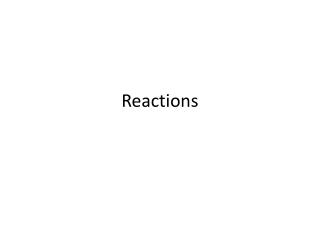DownloadDownload PresentationReactions

# Reactions

Télécharger la présentation## Reactions

- - - - - - - - - - - - - - - - - - - - - - - - - - - E N D - - - - - - - - - - - - - - - - - - - - - - - - - - -
##### Presentation Transcript

1. Reactions

2. Seven Diatomic Elements • (Super Seven) neverappear alonein nature. They are chemically combined in compounds or with themselves. These include hydrogen, nitrogen, oxygen, fluorine, chlorine, bromine, and iodine. • H2, N2, O2, F2­, Cl2, Br2, I2­

3. IV. Types of Chemical Reactions • Combination or Synthesis • A reaction in which two or more substance combine to form a single product • Examples:

4. 2. Examples: • 2H2 + O2 2 H2O • 4Al + 3O2 2Al2O3 • Ca + Cl2  CaCl2 • 2KCl + 3O2  2KClO3 • Notice that in each example, a compound is formed from elements or smaller compounds. This may be represented by the following general equation: A + B  AB

5. B. Decomposition Reaction • A reaction in which a compound is broken down into simpler substances such as elements or smaller compounds • Examples

6. 2. Examples • 2HgO 2Hg + O2 • 2H2O 2H2 + O2 • 2 KClO32KCl + 3O2 • Decomposition reactions are generally the opposite of composition reactions. Such a reaction may be represented by the following general equation: AB  A + B

7. C. Single Replacement Reaction • A reaction in which one element reacts with a compoundto form a differentelement and another compound • Examples

8. 2. Examples • Cu + 2AgNO3 Ag + Cu(NO3)2 • Zn + Cu(NO3)2 Cu + Zn(NO3)2 • Mg + 2HCl  H2 + ZnCl2 • These reactions occur according to the activity series of metals. Elements on the topof the list may replaceelements belowthem in a chemical reaction. For the Halogens, elements may replace those belowthem in the group. • The general equation for a single replacement reactions looks like: A + BC  B + AC

9. D. Double Replacement Reactions • A reaction in which the metals (or cations) present in two compounds changes places to form two new compounds. • Examples

10. 2. Examples • AgNO3 + NaClAgCl + NaNO3 • NaOH + HCl NaCl + H2O • Ba(NO3)2 + Na2SO4  BaSO4 + 2NaNO3 • The general equation for a double replacement reactions is: AB + CD  AD + CB • These reactions often use the solubility rules to predict precipitateformation.

11. E. Combustion Reaction • A reaction in which oxygenreacts with another substance usually producing heat and light. (We will be dealing primarily with the combustion of hydrocarbonfuels) • There are two kinds of combustions: complete combustion and incompletecombustion

12. Examples • CxHy + O2 CO2 + H2O • CxHy + O2 CO + C + H2O • Elemental carbon or soot, among other things may be formed during incomplete combustion.

13. F. Oxidation – Reduction Reactions • A reaction in which the oxidation number of an element changes. • Think of a number line. If the oxidation number moves to the right on the number line (becomes less negative or more positive) the element is oxidized. If the oxidation number moves to the lefton the number line (becomes more negative or less positive) the element is reduced. • To calculate the oxidation of the element, use the charge of the element or polyatomic ion to determine the charge of the metal in each substance. Elements in their natural state have an oxidation number of zero.

14. 4. Examples • Ag + NaClAgCl + Na • Al + Cu(NO3)2  Cu + Al(NO3)3 • For this course, we will concentrate on oxidation-reduction reactions that are also single replacement reactions, but others do exist.

15. What type of chemical reaction is represented by the following equations? • Ca + BaO Ba + CaO • 2C3H6+ 9O2 6CO2 + 6H2O • Ca+ O2CaO • Na3PO4+ BaCl2 Ba3(PO4)2 + NaCl • MgCl2 Mg + Cl2 • SR • CB • S • DR • D

16. Nuclear Reactions • A reaction in which nucleotides(particles) react during nuclear fusion or fission. • Fusion– is the combinationof two smaller nucleotides for form a larger, more massive particle. This is what happens in our sun. • Fission– is the splittingof a large, unstable nucleotide into smaller particles. This occurs in nuclear reactors.

17. The particles involved include the following • Alpha particles, a, look like helium ions: • Beta particles, b, look like electrons: • Positronslook like positive beta particles: • Neutronsare just neutrons: • Protonsare often written as hydrogen ions: • Gamma Rays – really a form of radiation that accompanies many nuclear reactions: or just g

18. To balance a nuclear reaction, make sure the total mass numbers on each side of the reaction are equal and the total atomic numbers on each side are equal. • Examples 6 + 2 = 4 + x x=4 3 + 1 = 2 + y y = 2

19. _____ • _____

20. 7. Radiation • Alpha radiation is the least penetrating. It can be stopped by a piece of paper. This is because of the size of the particles. • Beta radiation is moderately penetrating. Aluminum foil is thick enough to stop beta radiation. • Gamma radiation is the most penetrating because it is a form of electromagnetic radiation. Layers of concrete and lead are needed to stop gamma radiation.